# Guessing Passwords using an LSTM Network

## Introduction

A while ago I applied NLP strategies to implement an algorithm that is capable to guess a password. Since then a new method called transformer was developed and successfully used in many NLP tasks. Before transformers were the method of choice, LSTM Models dominated the field. In this article we will lay down a setup for such an LSTM Model to guess passwords and derive why a network might be better suited than the Markov chain model presented in this post.

A shot recap of our notation: Letbe a password and the random variables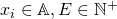the corresponding letters and password length.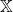is the space of all passwords andcorresponds to the used alphabet, for example if we allow only for lower case letters then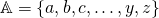. As usual this forms a discrete probability space given by. The task to solve is to derive.

In the Markov chain example it is assumed that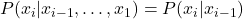which obviously falls short. Our strategy to overcome this problem was to use “-grams”, therefore one can look at sequences of lengthinstead of a single letter. However with raisinga major problem is that the possibility to observe a each sequence in the training set decreases which will lead to an underestimation of the probability for such sequences. This is where Recurrent Neuronal Networks (RNN) step in (LSTM is a specific RNN).

## The terminology of neuronal networks

To start with, we will first have a brief look at more simple networks. There has been a great deal of hype surrounding
neural networks, making them seem magical and mysterious. However, they are just nonlinear statistical models . In particular, a neuronal network is a class of functions mapping a random variable. However the terminology in the literature is quiet different.  gave the following translation:

• variables are called features
• independent variables are called inputs
• predicted values are called outputs
• dependent variables are called targets or training values
• residuals are called errors
• estimation is called training, learning, adaptation, or self-organization
• an estimation criterion is called an error function, cost function, or Lyapunov function
• observations are called patterns or training pairs
• parameter estimates are called (synaptic) weights
• interactions are called higher-order neurons
• transformations are called functional links
• regression and discriminant analysis are called supervised learning or heteroassociation
• data reduction is called unsupervised learning, encoding, or autoassociation
• cluster analysis is called competitive learning or adaptive vector quantization

The statistical terms sample and population do not seem to have Neuronal Network equivalents. However, the data are often divided into a training set and test set for cross-validation.

## Feed-Forward Neuronal Networks

Our next work guessing task can be understood like as a classification problem where the number of classes corresponds to the size of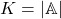. Our target in this case is to predict the actual letterbased on the previously observed letter. Since neuronal nets can not deal with letters directly we have to convert them into an input vectorwithcomponents and a target vectorwhich has alsocomponents (in general the size of the vectors can differ). Both vectors are dummy variables (one-hot encoding) such that the-th component ofisif the observed letteris at the-th position of the alphabetandelse.
Additionally we construct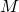derived features, these features can be compared to a linear basis generated from the data.is called the hidden layer, since the outputs of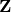are not directly observed. Adding more then one hidden layer enables the network to capture interactions. Thus each componentfor the estimatorofis modeled byas a linear combination of:where. A popular choice for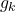is the softmax functionwhich ensures thatis between [0,1]. In a regression setup frequentlyis used.is an “activation function, popular choices areor the sigmoid function.
The system of equations has unknown variableswhere,to be estimated from the data.
Assume that we observepasswords with lengthresulting in pairs consisting of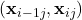. To fit the network we have to define a suitable loss function, since we deal with a classification problem we use the cross-entropy loss functionminimized over.

## Recurrent Neuronal Networks and LSTM

By construction the Feed-Forward network suffers from the same issue as Markov-Chain attempt, we only look at the previous letter ignoring the entire prior sequence. To model such time dependent sequences RNN steps in. We will therefore extend our network, allowing to use previous letters of the password sequences by altering the hidden layer such that an estimator foris given by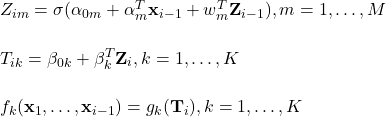This model now consists of unknown variables,,to be estimated from the data. Assume again that we observepasswords with length. To train the network we use all possible subsequences,, … .

An RNN works well to handle sequential data but runs into problems if influential letters are far away. This is not much a problem of the network itself, but rather of the methods used to train it. Neuronal Networks where fitted using backpropagation, the problem concerning RNN are vanishing/exploding gradients  LSTM (to a major part) solves this issue by allowing to connect letters which are far away. This is archived with having a shortcut into connect the LSTM cells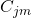of different letters .In the literature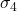andare represented by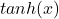, while the choice of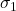tois usually a sigmoid function.

## Results

The proposed methods where used to fit a Feedforward, a Recurrent Neuronal net as well as a Long-Short-Term-Memory net. To train the network the 10.000.000 most popular passwords where used. The fitted models can be found in my github repo the corresponding code can be found here. Figure 1-3 represent the probabilities for the next letter if “lov” was observed. We can see that while LSTM and RNN predict “e” as the most probable letter the Feedforward net prefers “eof” which means that the passwords ends. This misjudgment is not surprising, since all passwords have in common that they end at some point. Because the FF network does not know the length of the password, the best guess is the end of the password. RNN and LSTM do not make this mistake. It is noticeable that the probabilities look similar, but in the LSTM network the probabilities concerning “e” are more pronounced.

 T. Hastie, R. Tibshirani, and J. Friedman, The elements of statistical learning, New York, NY, USA: Springer new york inc., 2001.
[Bibtex]
@book{hastie01statisticallearning,
address = {New York, NY, USA},
author = {Hastie, Trevor and Tibshirani, Robert and Friedman, Jerome},
interhash = {d585aea274f2b9b228fc1629bc273644},
keywords = {ml statistics},
publisher = {Springer New York Inc.},
series = {Springer Series in Statistics},
timestamp = {2008-05-16T16:17:43.000+0200},
title = {The Elements of Statistical Learning},
year = 2001
}
 W. Sarle, “Neural networks and statistical models.” 1994.
[Bibtex]
@inproceedings{Sarle1994NeuralNA,
title={Neural Networks and Statistical Models},
author={Warren Sarle},
year={1994}
}
 S. Hochreiter and J. Schmidhuber, “Long short-term memory,” Neural computation, vol. 9, pp. 1735-1780, 1997.
[Bibtex]
@article{Hochreiter1997LongSM,
title={Long Short-Term Memory},
author={Sepp Hochreiter and J{\"u}rgen Schmidhuber},
journal={Neural Computation},
year={1997},
volume={9},
pages={1735-1780}
}

This site uses Akismet to reduce spam. Learn how your comment data is processed.